### ReferenceExpressions

#### Introduction

This page presents a collection of expressions that can be used with source code for surface and displacement shaders. The expressions use the values of the global variables s and t, both of which by default are in the range 0 to 1, to calculate values in other ranges.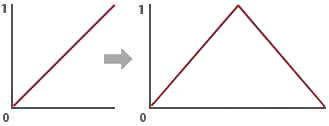Figure 1

Being able to conveniently convert one range of values into another range is very useful when, for example, the effect of a surface or displacement shader needs to be gradually modified towards the edges of a surface.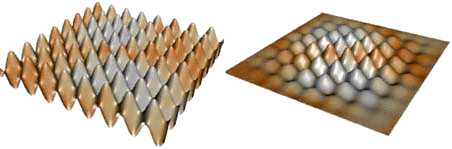Figure 2

#### Mapping 0 - 1 to 1 - 0 - 1

Deriving an expression that will convert values in one range to their associated values in another range can be visualized as a process of 'moving' and 'stretching' the initial range, say 0 to 1, along a fixed number line.Figure 3

For example, the diagram below shows the original range, 0 to 1, being mapped to the range 1 to 0 to 1.Figure 4

If the original range of values was represened by say the s texture coordinate, new values could be calculated using the following expression.
`    newValue = abs((s - 0.5) * 2);`

The effect of using newValue to scale the displacement of a wave is shown below.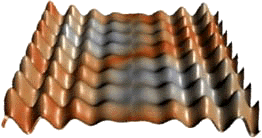Figure 5

#### Mapping 0 - 1 to 0 - 1 - 0

The diagram below shows the original range, 0 to 1, being mapped to the range 0 to 1 to 0.Figure 6

The equivalent expression is,
`    newValue = abs (abs(s - 0.5) * 2 - 1);`

The effect of using newValue, calculated with both 's' and 't', to scale the displacement of a turbulance shader is shown below.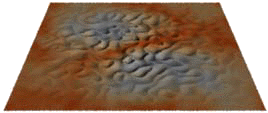Figure 7

#### Mapping a Subrange to 0 - 1 - 0

The diagrams show a subrange a to c being mapped to the range 0 to 1 to 0. It is assumed b is the midpoint of acFigure 8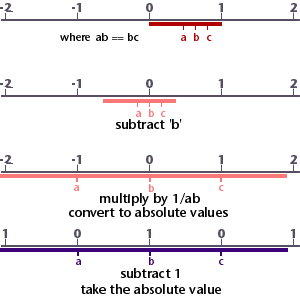Figure 9

The equivalent expression is,
`    newValue = abs (abs((s - b) * 1/ab) - 1);`

The effect of using this expression to calculate a displacement in both 's' and 't' is shown below.Figure 10

#### Using the Sine Function

The sine function is part of the maths library that is built into the shading language. The function returns a value that is the ratio of the length of a rotating line divided by its vertical height. The end of the rotating line sweeps out the circumference of a circle. If the line is one unit long the circumference of the circle is 2 x PI or 6.283185 units in length.Figure 11

In the diagram shown above the vertical height of the rotating line, itself 1 unit in length, can never be greater than +1 and never be less than -1. Consequently for any rotation of the line, measured by the distance its end has moved along the circumference of the circle, the sine function returns values in the range +1 to -1. The unit of measurement used to express the rotation is the radian.

To generate the sine wave shown above across a surface, say in the 's' direction, we must scale its value by 2PI ie. multiply by 6.283185.
`    waveHeight = sin (s * 6.283185);`

The effect of using waveHeight in a displacement shader is shown below.Figure 12

To avoid negative numbers it is often necessary to keep waveHeight in the range 0 to 1. The following expression accomplishes this,
`    waveHeight = (sin(s * 6.283185) + 1) * 0.5;`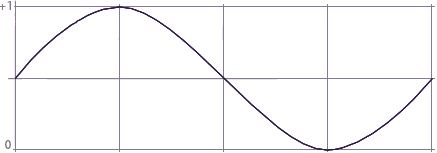Figure 13

It is also useful to be able to shift the sine wave horizonally so that the wave begins and ends at a 'trough', a 'peak' or anywhere in between. The following expression introduces control over the phase of the wave.
`    waveHeight = (sin ((s - phase) * 6.283185) + 1.0) * 0.5;`
when "phase" is 1.0 the wave is shifted one complete cycle.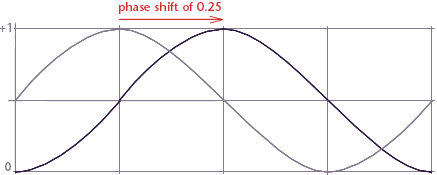Figure 14

Often an arbitary number of cycles of the sine wave is required, the following expression includes a variable that controls the number of waves,
`    waveHeight = (sin((s-phase/cycles)*6.283185*cycles) + 1.0)*0.5;`Figure 15

The effect of using this expression in a displacement shader is shown below.Figure 16

Occasionally it is necessary to modify the sine wave so that it produces only 'bumps'. Here is the expression,
`    waveHeight = abs (sin ((s - phase/cycles) * 6.282 * cycles));`
Used in a displacement shader this expression produces the effect shown below.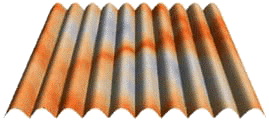Figure 17

#### Using the Mod Function

The mod function divides one number by another but returns only the remainder. For example,
`    mod (3, 2);`
would return the value 0.5, not 1.5.
The function can be used to map the range 0 to 1 to a sequence of repeating sub-ranges 0 to ~1. In each sub-range the maximum value becomes infinitly close to 1 but never actually reaches that value.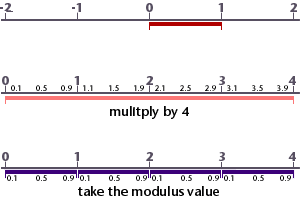Figure 18

The mod function is very useful when creating repeating patterns on a surface. In the diagram above, 4 sub-ranges have been created, however, the function can be used to make any number of repeats. To generate 8 repeated patterns, say in the 's' direction, the following expression would be applied.
`    ss = mod (s * 8, 1);`
Using 1 as the divider ensures the number of 'repeats' is not altered. The effect of using mod in a displacement shader, in both the 's' and 't' directions is shown below.Figure 19